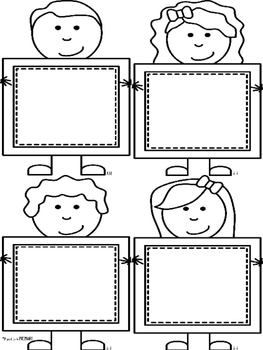# Number Line Math Game

Rated 4.71 out of 5, based on 122 reviews
122 Ratings;
K - 2nd
Subjects
Resource Type
Standards
Formats Included
• PDF
Pages
17 pages
Report this resource to TPT

### Description

This human number line math game features 24 math flash cards with 3 number addition, subtraction or mixed equations.

Also included are the colored numbers 1-9 for you to label your number line.

There is a blank flash card sheet for you to use for your own games as a bonus!

Full step by step instructions are provided in this freebie with photographs for you to see how I used this in my classroom! Read the blog post HERE for tips.

You might also be interested in:

Mindful Math - A Complete First Grade Curriculum

Daily Math Mats

Math Workshop Tool Kit

Math In A Minute - Addition Fact Practice

Math In A Minute - Subtraction Fact Practice

Stay connected with Proud to be Primary

♥Visit our website at Proud to be Primary for tons of engaging ideas for teaching kids in the classroom!

Questions, suggestions, and feedback are always welcome.

Just use the “Product Q&A” tab and I will get back to you as fast as I can!

Total Pages
17 pages
N/A
Teaching Duration
1 month
Report this resource to TPT
Reported resources will be reviewed by our team. Report this resource to let us know if this resource violates TPT’s content guidelines.

### Standards

to see state-specific standards (only available in the US).
Relate counting to addition and subtraction (e.g., by counting on 2 to add 2).
Add and subtract within 20, demonstrating fluency for addition and subtraction within 10. Use strategies such as counting on; making ten (e.g., 8 + 6 = 8 + 2 + 4 = 10 + 4 = 14); decomposing a number leading to a ten (e.g., 13 - 4 = 13 - 3 - 1 = 10 - 1 = 9); using the relationship between addition and subtraction (e.g., knowing that 8 + 4 = 12, one knows 12 - 8 = 4); and creating equivalent but easier or known sums (e.g., adding 6 + 7 by creating the known equivalent 6 + 6 + 1 = 12 + 1 = 13).
Represent addition and subtraction with objects, fingers, mental images, drawings, sounds (e.g., claps), acting out situations, verbal explanations, expressions, or equations.
Solve addition and subtraction word problems, and add and subtract within 10, e.g., by using objects or drawings to represent the problem.